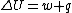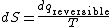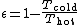thermodynamic principles

Thermodynamic principles

Laws governing the transformation of energy. Thermodynamics is the science of the transformation of energy. It differs from the dynamics of Newton by taking into account the concept of temperature, which is outside the scope of classical mechanics. In practice, thermodynamics is useful for assessing the efficiencies of heat engines (devices that transform heat into work) and refrigerators (devices that use external sources of work to transfer heat from a hot system to cooler sinks), and for discussing the spontaneity of chemical reactions (their tendency to occur naturally) and the work that they can be used to generate.

The subject of thermodynamics is founded on four generalizations of experience, which are called the laws of thermodynamics. Each law embodies a particular constraint on the properties of the world. The connection between phenomenological thermodynamics and the properties of the constituent particles of a system is established by statistical thermodynamics, also called statistical mechanics. Classical thermodynamics consists of a collection of mathematical relations between observables, and as such is independent of any underlying model of matter (in terms, for instance, of atoms). However, interpretations in terms of the statistical behavior of large assemblies of particles greatly enriches the understanding of the relations established by thermodynamics. See Statistical mechanics

Zeroth law of thermodynamics

The zeroth law of thermodynamics establishes the existence of a property called temperature. This law is based on the observation that if a system A is in thermal equilibrium with a system B (that is, no change in the properties of B take places when the two are in contact), and if system B is in thermal equilibrium with a system C, then it is invariably the case that A will be found to be in equilibrium with C if the two systems are placed in mutual contact. This law suggests that a numerical scale can be established for the common property, and if A, B, and C have the same numerical values of this property, then they will be in mutual thermal equilibrium if they were placed in contact. This property is now called the temperature. See Temperature

First law of thermodynamics

The first law of thermodynamics establishes the existence of a property called the internal energy of a system. It also brings into the discussion the concept of heat.

The first law is based on the observation that a change in the state of a system can be brought about by a variety of techniques. Indeed, if attention is confined to an adiabatic system, one that is thermally insulated from its surroundings, then the work of J. P. Joule shows that same change of state is brought about by a given quantity of work regardless of the manner in which the work is done. This observation suggests that, just as the height through which a mountaineer climbs can be calculated from the difference in altitudes regardless of the path the climber takes between two fixed points, so the work, w, can be calculated from the difference between the final and initial properties of a system. The relevant property is called the internal energy, U. However, if the transformation of the system is taken along a path that is not adiabatic, a different quantity of work may be required. The difference between the work of adiabatic change and the work of nonadiabatic change is called heat, q. In general, Eq. (1)

(1)is satisfied, where ΔU is the change in internal energy between the final and initial states of the system. See Adiabatic process, Energy, Heat

The implication of this argument is that there are two modes of transferring energy between a system and its surroundings. One is by doing work; the other is by heating the system. Work and heat are modes of transferring energy. They are not forms of energy in their own right. Work is a mode of transfer that is equivalent (if not the case in actuality) to raising a weight in the surroundings. Heat is a mode of transfer that arises from a difference in temperature between the system and its surroundings. What is commonly called heat is more correctly called the thermal motion of the molecules of a system.

The first law of thermodynamics states that the internal energy of an isolated system is conserved. That is, for a system to which no energy can be transferred by the agency of work or of heat, the internal energy remains constant. This law is a cousin of the law of the conservation of energy in mechanics, but it is richer, for it implies the equivalence of heat and work for bringing about changes in the internal energy of a system (and heat is foreign to classical mechanics).

Second law of thermodynamics

The second law of thermodynamics deals with the distinction between spontaneous and nonspontaneous processes. A process is spontaneous if it occurs without needing to be driven. In other words, spontaneous changes are natural changes, like the cooling of hot metal and the free expansion of a gas. Many conceivable changes occur with the conservation of energy globally, and hence are not in conflict with the first law; but many of those changes turn out to be nonspontaneous, and hence occur only if they are driven.

The second law was formulated by Lord Kelvin and by R. Clausius in a manner relating to observation: “no cyclic engine operates without a heat sink” and “heat does not transfer spontaneously from a cool to a hotter body,” respectively (see illustration). The two statements are logically equivalent in the sense that failure of one implies failure of the other. However, both may be absorbed into a single statement: the entropy of an isolated system increases when a spontaneous change occurs. The property of entropy is introduced to formulate the law quantitatively in exactly the same way that the properties of temperature and internal energy are introduced to render the zeroth and first laws quantitative and precise.

The entropy, S, of a system is a measure of the quality of the energy that it stores. The formal definition is based on

(2)Eq. (2), where dS is the change in entropy of a system, dq is the energy transferred to the system as heat, T is the temperature, and the subscript “reversible” signifies that the transfer must be carried out reversibly (without entropy production other than in the system). When a given quantity of energy is transferred as heat, the change in entropy is large if the transfer occurs at a low temperature and small if the temperature is high.

This definition of entropy is illuminated by L. Boltzmann's interpretation of entropy as a measure of the disorder of a system. The connection can be appreciated qualitatively at least by noting that if the temperature is high, the transfer of a given quantity of energy as heat stimulates a relatively small additional disorder in the thermal motion of the molecules of a system; in contrast, if the temperature is low, the same transfer could stimulate a relatively large additional disorder.

The illumination of the second law brought about by the association of entropy and disorder is that in an isolated system the only changes that may occur are those in which there is no increase in order. Thus, energy and matter tend to disperse in disorder (that is, entropy tends to increase), and this dispersal is the driving force of spontaneous change. See Entropy, Time, arrow of

Third law of thermodynamics

The practical significance of the second law is that it limits the extent to which the internal energy may be extracted from a system as work. In order for a process to generate work, it must be spontaneous. For the process to be spontaneous, it is necessary to discard some energy as heat in a sink of lower temperature. In other words, nature in effect exacts a tax on the extraction of energy as work. There is therefore a fundamental limit on the efficiency of engines that convert heat into work.

The quantitative limit on the efficiency, ε, which is defined as the work produced divided by the heat absorbed from the hot source, was first derived by S. Carnot. He found that, regardless of the details of the construction of the engine, the maximum efficiency (that is, the work obtained after payment of the minimum allowable tax to ensure spontaneity) is given by Eq. (3),

(3)where Thot is the temperature of the hot source and Tcold is the temperature of the cold sink. The greatest efficiencies are obtained with the coldest sinks and the hottest sources, and these are the design requirements of modern power plants.

Perfect efficiency (ε = 1) would be obtained if the cold sink were at absolute zero (Tcold = 0). However, the third law of thermodynamics, which is another summary of observations, asserts that absolute zero is unattainable in a finite number of steps for any process. Therefore, heat can never be completely converted into work in a heat engine. The implication of the third law in this form is that the entropy change accompanying any process approaches zero as the temperature approaches zero. That implication in turn implies that all substances tend toward the same entropy as the temperature is reduced to zero. It is therefore sensible to take the entropy of all perfect crystalline substances (substances in which there is no residual disorder arising from the location of atoms) as equal to zero. A common short statement of the third law is therefore that all perfect crystalline substances have zero entropy at absolute zero (T = 0). This statement is consistent with the interpretation of entropy as a measure of disorder, since at absolute zero all thermal motion has been quenched. See Absolute zero

thermodynamic principles

[¦thər·mō·dī′nam·ik ′prin·sə·pəlz]
(thermodynamics)
Laws governing the conversion of energy from one form to another.
References in periodicals archive ?
Frank Diederichs from Oasis Publishers has done outstanding research on Heat Production in Skeletal muscles using Chemical Thermodynamic Principles.
With the exception of a few investigators, none has considered the solubility of MnS (from thermodynamic principles) as a guide to balance manganese and sulfur.
The collaborators described their research - including the thermodynamic principles behind it - in a paper that was delivered at the IMechE Vehicle Thermal Management Systems Conference in London.
The story progresses briskly through Vilhelm Bjerknes's early work on hydrodynamics, his progression from ideal fluids to real fluids including the atmosphere and ocean, to his setting out in Meteorologische Zeitschrift in 1902 a method of calculating the future state of the weather based upon sound hydrodynamic and thermodynamic principles. It was not all theory, of course, and Fleming describes the establishment of the "Bergen forecasting school" in 1917, founded initially to ensure Norway was able to feed its people during the long years of World War I by providing improved short-term forecasts for the country's vital agricultural and fishing sectors.
Any future practical applications of this work will depend on understanding how basic thermodynamic principles operate at ultrasmall scales.
During his study of basic thermodynamic principles, he derived a mathematical equation to determine a jet's level of maneuverability based on basic information like thrust rating, aerodynamic drag, lift coefficients, and aircraft weight.
The proxy relies on thermodynamic principles, taking advantage of the temperature-dependence of the binding strength between different isotopes of carbon and oxygen, which makes it independent of other variables.
Section 3 treats subjects related to refractories, such as classification, thermodynamic principles, properties and testing, production, silica, alumina, alumino-silicate, chrome-magnetite, and carbon.
Several aspects demonstrate Whittle's complete understanding of the thermodynamic principles and the challenges facing the designer.
Al-Shemmeri (renewable energy technology, Staffordshire U.) tackles the fundamental principles of thermodynamics in day-to-day engineering concepts, provides the tools to measure process efficiency and sustainability accurately in power and heating applications, and helps engineers recognize why losses occur and how they can be reduced by applying familiar thermodynamic principles. Worked examples and tutorial problems are included for classroom use or self-study.
Beavers focuses on Bigger as a figuration of surplus meaning, as he interprets the novel as a work that reveals thermodynamic principles.
The objective of the course is to provide the practicing engineers in power generation industry with fundamental thermodynamic principles that govern the design and performance of Gas Turbine Combined Cycle power plants.

Site: Follow: Share:
Open / Close